# Investigation of impact motions in elements of manipulators and robots

## K. Ragulskis1, B. Spruogis2, A. Matuliauskas3, V. Mištinas4, L. Ragulskis5

1Kaunas University of Technology, K. Donelaičio str. 73, LT-44249, Kaunas, Lithuania

2, 3, 4Department of Mobile Machinery and Railway Transport, Faculty of Transport Engineering, Vilnius Gediminas Technical University, Plytinės str. 27, LT-10105, Vilnius, Lithuania

5Department of Systems Analysis, Faculty of Informatics, Vytautas Magnus University, Vileikos str. 8, LT-44404, Kaunas, Lithuania

1Corresponding author

Mathematical Models in Engineering, Vol. 7, Issue 3, 2021, p. 54-62. https://doi.org/10.21595/mme.2021.22240
Received 7 July 2021; received in revised form 19 September 2021; accepted 27 September 2021; published 30 September 2021

Copyright © 2021 K. Ragulskis, et al. This is an open access article distributed under the Creative Commons Attribution License, which permits unrestricted use, distribution, and reproduction in any medium, provided the original work is properly cited.
Views 39
CrossRef Citations 0
Abstract.

Impact interactions take place in the process of dynamic behavior in the elements of manipulators and robots. Their precise investigation is an important engineering problem. A model having one degree of freedom with forced excitation and impacts is investigated. A special procedure for more precise calculation of impacts is proposed. Numerical results for various parameters of the investigated system are presented. The advantage of the application of the proposed procedure for more precise calculations of dynamics of this system is indicated.

Keywords: elements of manipulators, elements of robots, impact interactions, forced excitation, nonlinear behaviour, graphical representations.

#### 1. Introduction

Impact interactions take place in the process of dynamic behavior in the elements of manipulators and robots. Their precise investigation is an important engineering problem.

A model having one degree of freedom with forced excitation and impacts is investigated. A special procedure for more precise calculation of impacts is proposed. Numerical results for various parameters of the investigated system are presented. The advantage of the application of the proposed procedure for more precise calculations of dynamics of this system is indicated.

Basic investigations of vibrations of vibro impact systems are presented in . Vibrations of transmissions and nonlinear effects in them are investigated in . Dynamics of vibromotors and their applications are described in . This paper is based on the results of those three fundamental references.

Nonlinear systems as well as resonances in them are investigated in . Systems with various types of excitations and impacts are analysed in . Nonlinear dynamical systems and their stabilisation are described in . Impacts and dynamics of vibrating systems are analysed in . Mechanical systems and their periodic orbits are investigated in . Nonlinear qualities of vibro-impact energy sink are described in . Interaction of particle and a wall is analysed in . Multibody dynamical system and its frequencies are investigated in . Dynamics of a pendulum in a mechanism is analysed in . Dynamical system with piecewise linearity is investigated in . Vibrating system and its resonant zones are analysed in . Dynamical system with Sommerfeld effect is investigated in . Dynamical system with isolated resonances is analysed in .

In this paper the model of the investigated system is described and then the proposed procedure for more precise calculations of impacts is presented. Results for various parameters of the investigated system without application of the proposed procedure and with it are presented.

#### 2. Model of the vibro impact system with forced excitation

The investigated vibro impact system is described by the following equation:

(1)
$\stackrel{¨}{x}+2h\stackrel{˙}{x}+{p}^{2}x=-f\mathrm{s}\mathrm{i}\mathrm{n}\omega t,$

where $x$ denotes the displacement of the investigated system, $h$ is the coefficient of viscous damping of the investigated system, $p$ is the eigenfrequency of the undamped system, $f$ is the amplitude of the exciting force, $\omega$ is the frequency of the exciting force, $t$ is the time variable and the upper dot denotes differentiation with respect to the time.

When the following conditions are satisfied:

(2)
$x\ge 0,$

and

(3)
$\stackrel{˙}{x}>0,$

then it is assumed that:

(4)
$x=0,$

and

(5)
${\stackrel{˙}{x}}^{+}=-R{\stackrel{˙}{x}}^{-},$

where $R$ is the coefficient of restitution and the superscript minus indicates the value before the impact, while the superscript plus indicates the value after the impact.

#### 3. Procedure for improved calculation of impacts in the investigated system

Further $T$ denotes the time step, the subscript $0$ indicates the value at the beginning of a time step and the subscript $T$ indicates the value at the end of a time step.

When the following condition is satisfied:

(6)
${x}_{T}>0,$

then the reduced time step is defined as:

(7)
${T}_{r}=T\frac{0-{x}_{0}}{{x}_{T}-{x}_{0}}.$

Then it is assumed that:

(8)
${x}_{{T}_{r}}=0,$
(9)
${\stackrel{˙}{x}}_{{T}_{r}}={\stackrel{˙}{x}}_{0}+\left({\stackrel{˙}{x}}_{T}-{\stackrel{˙}{x}}_{0}\right)\frac{{T}_{r}}{T},$
(10)
${\stackrel{¨}{x}}_{{T}_{r}}={\stackrel{¨}{x}}_{0}+\left({\stackrel{¨}{x}}_{T}-{\stackrel{¨}{x}}_{0}\right)\frac{{T}_{r}}{T}.$

#### 4. Results of investigations of dynamics of the vibro impact system with forced excitation

The following values of the parameters of the vibro impact system with forced excitation were assumed in the presented investigation:

(11)

Zero initial conditions were assumed, that is:

(12)

The following time step was used:

(13)
$T=\frac{\frac{2\pi }{\omega }}{100}.$

Investigations were performed for three sets of parameters of coefficient of viscous damping and coefficient of restitution: 1) conservative impacts, 2) conservative inter impact motions, 3) fully dissipative behaviour.

#### 4.1. Results of investigation of dynamics of the vibro impact system with forced excitation for the case of conservative impacts

The following values of the parameters of the vibro impact system with forced excitation were assumed in the presented investigation:

(14)

Graphical results of investigation of dynamics of the vibro impact system with forced excitation without application of the procedure for improved calculation of impacts are presented in Fig. 1.

Fig. 1. Dynamics of the vibro impact system with forced excitation without application of the procedure for improved calculation of impacts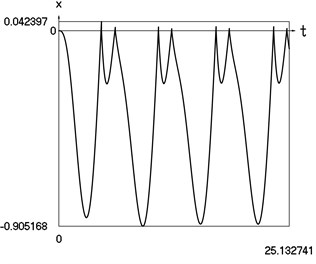a) Displacement as function of time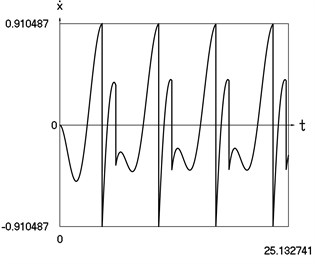b) Velocity as function of time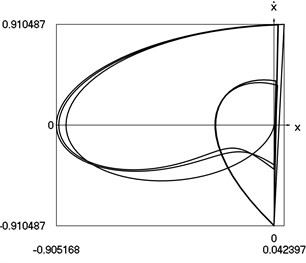c) Representation in the phase plane: velocity as function of displacement

In Fig. 1 displacement as function of time, velocity as function of time and representation of dynamics of the investigated system in the phase plane are presented.

Graphical results of investigation of dynamics of the vibro impact system with forced excitation with application of the procedure for improved calculation of impacts are presented in Fig. 2.

Fig. 2. Dynamics of the vibro impact system with forced excitation with application of the procedure for improved calculation of impacts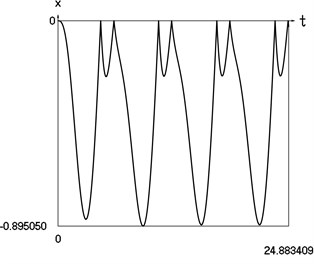a) Displacement as function of time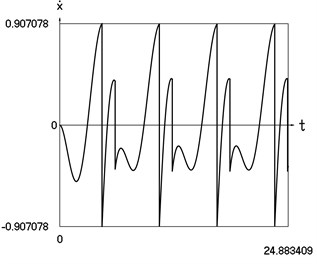b) Velocity as function of time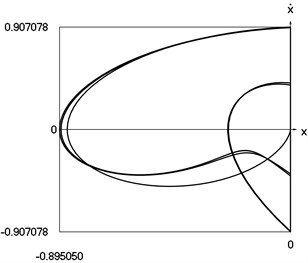c) Representation in the phase plane: velocity as function of displacement

In Fig. 2 displacement as function of time, velocity as function of time and representation of dynamics of the investigated system in the phase plane are presented.

From the comparison of graphical results presented in Fig. 1 and Fig. 2 it is seen that the procedure of improved calculation of impacts has advantages especially evident in the suppression of small positive values of displacements seen in the process of mutual comparison of drawings a) and c) from those figures.

#### 4.2. Results of investigation of dynamics of the vibro impact system with forced excitation for the case of conservative inter impact motions

The following values of the parameters of the vibro impact system with forced excitation were assumed in the presented investigation:

(15)

Graphical results of investigation of dynamics of the vibro impact system with forced excitation without application of the procedure for improved calculation of impacts are presented in Fig. 3.

In Fig. 3 displacement as function of time, velocity as function of time and representation of dynamics of the investigated system in the phase plane are presented.

Fig. 3. Dynamics of the vibro impact system with forced excitation without application of the procedure for improved calculation of impacts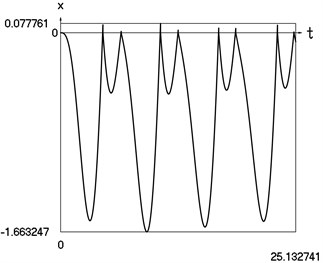a) Displacement as function of time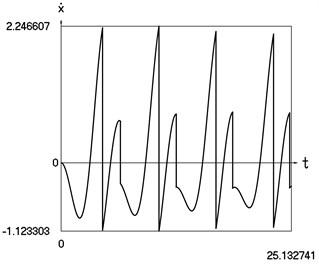b) Velocity as function of time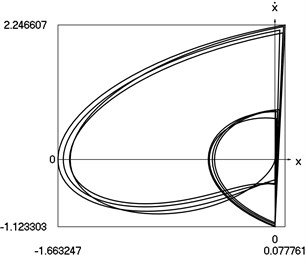c) Representation in the phase plane: velocity as function of displacement

Fig. 4. Dynamics of the vibro impact system with forced excitation with application of the procedure for improved calculation of impacts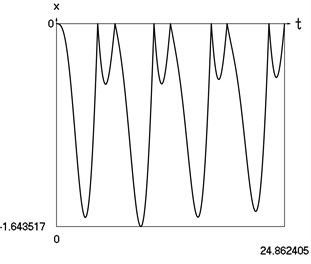a) Displacement as function of time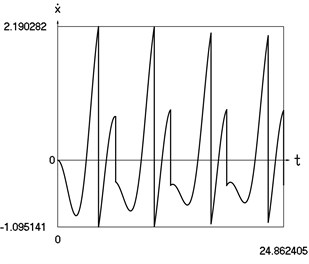b) Velocity as function of time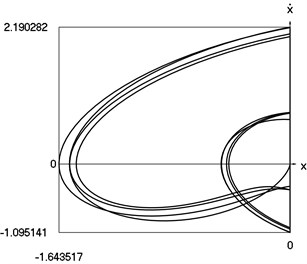c) Representation in the phase plane: velocity as function of displacement

Graphical results of investigation of dynamics of the vibro impact system with forced excitation with application of the procedure for improved calculation of impacts are presented in Fig. 4.

In Fig. 4 displacement as function of time, velocity as function of time and representation of dynamics of the investigated system in the phase plane are presented.

From the comparison of graphical results presented in Fig. 3 and Fig. 4 it is seen that the procedure of improved calculation of impacts has advantages especially evident in the suppression of small positive values of displacements seen in the process of mutual comparison of drawings a) and c) from those figures.

#### 4.3. Results of investigation of dynamics of the vibro impact system with forced excitation for the case of fully dissipative behaviour

The following values of the parameters of the vibro impact system with forced excitation were assumed in the presented investigation:

(16)

Graphical results of investigation of dynamics of the vibro impact system with forced excitation without application of the procedure for improved calculation of impacts are presented in Fig. 5.

Fig. 5. Dynamics of the vibro impact system with forced excitation without application of the procedure for improved calculation of impacts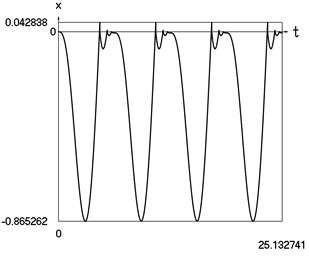a) Displacement as function of time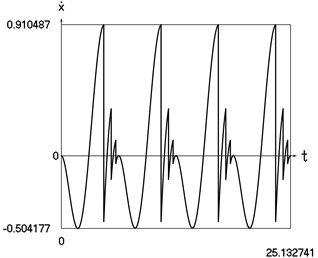b) Velocity as function of time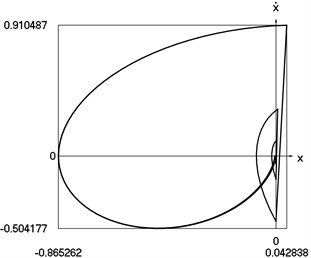c) Representation in the phase plane: velocity as function of displacement

In Fig. 5 displacement as function of time, velocity as function of time and representation of dynamics of the investigated system in the phase plane are presented.

Graphical results of investigation of dynamics of the vibro impact system with forced excitation with application of the procedure for improved calculation of impacts are presented in Fig. 6.

Fig. 6. Dynamics of the vibro impact system with forced excitation with application of the procedure for improved calculation of impacts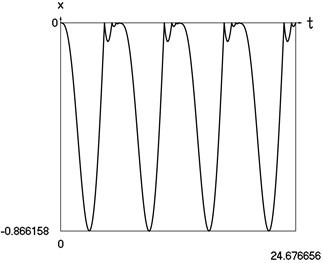a) Displacement as function of time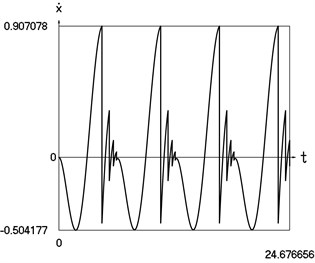b) Velocity as function of time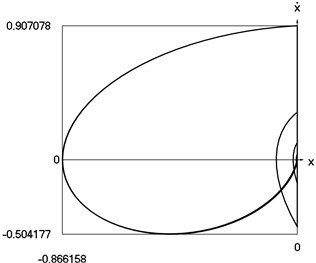c) Representation in the phase plane: velocity as function of displacement

In Fig. 6 displacement as function of time, velocity as function of time and representation of dynamics of the investigated system in the phase plane are presented.

From the comparison of graphical results presented in Fig. 5 and Fig. 6 it is seen that the procedure of improved calculation of impacts has advantages especially evident in the suppression of small positive values of displacements seen in the process of mutual comparison of drawings a) and c) from those figures.

From the presented graphical results advantages of the proposed procedure for calculation of impact motions are seen.

#### 5. Conclusions

The model of the investigated system is described and then the proposed procedure for more precise calculations of impacts is presented. Results for various parameters of the investigated system without application of the proposed procedure and with it are presented.

Investigations were performed for three sets of parameters of coefficient of viscous damping and coefficient of restitution: conservative impacts, conservative inter impact motions, fully dissipative behaviour. Displacement as function of time, velocity as function of time and representation of dynamics of the investigated system in the phase plane are investigated.

The advantage of the application of the proposed procedure for more precise calculations of dynamics of this vibro impact system with forced excitation is indicated and it is seen from comparisons of some of the graphical results presented which were obtained without application of the proposed procedure and with it. From the comparison of graphical results it is seen that the procedure of improved calculation of impacts has advantages especially evident in the suppression of small positive values of displacements seen in the process of mutual comparison of drawings obtained without application of the proposed procedure and with application of this procedure. From the presented graphical results advantages of the proposed procedure for calculation of impact motions are seen.

The obtained results are used in the process of design of elements of manipulators and robots in which impact interactions take place.

1. Ragulskienė V., Vibro-Shock Systems (Theory and Applications). (in Russian), Vilnius: Mintis, 1974. [Search CrossRef]
2. Kurila R. and Ragulskienė V., Two-Dimensional Vibro-Transmissions. (in Russian), Vilnius: Mokslas, 1986. [Search CrossRef]
3. Ragulskis K., Bansevičius R., Barauskas R., and Kulvietis G., Vibromotors for Precision Microrobots. New York: Hemisphere, 1987. [Search CrossRef]
4. Wedig W. V., “New resonances and velocity jumps in nonlinear road-vehicle dynamics,” Procedia IUTAM, Vol. 19, pp. 209–218, 2016. [Publisher]
5. Li T., Gourc E., Seguy S., and Berlioz A., “Dynamics of two vibro-impact nonlinear energy sinks in parallel under periodic and transient excitations,” International Journal of Non-Linear Mechanics, Vol. 90, pp. 100–110, Jan. 2017, https://doi.org/10.1016/j.ijnonlinmec.2017.01.010 [Publisher]
6. V. A. Zaitsev, “Global asymptotic stabilization of periodic nonlinear systems with stable free dynamics,” Systems and Control Letters, Vol. 91, pp. 7–13, May 2016, https://doi.org/10.1016/j.sysconle.2016.01.004 [Publisher]
7. H. Dankowicz and E. Fotsch, “On the analysis of chatter in mechanical systems with impacts,” Procedia IUTAM, Vol. 20, pp. 18–25, 2017, https://doi.org/10.1016/j.piutam.2017.03.004 [Publisher]
8. Spedicato S. and Notarstefano G., “An optimal control approach to the design of periodic orbits for mechanical systems with impacts,” Nonlinear Analysis: Hybrid Systems, Vol. 23, pp. 111–121, 2017. [Publisher]
9. W. Li, N. E. Wierschem, X. Li, and T. Yang, “On the energy transfer mechanism of the single-sided vibro-impact nonlinear energy sink,” Journal of Sound and Vibration, Vol. 437, pp. 166–179, Dec. 2018, https://doi.org/10.1016/j.jsv.2018.08.057 [Publisher]
10. J. S. Marshall, “Modeling and sensitivity analysis of particle impact with a wall with integrated damping mechanisms,” Powder Technology, Vol. 339, pp. 17–24, Nov. 2018, https://doi.org/10.1016/j.powtec.2018.07.097 [Publisher]
11. E. Salahshoor, S. Ebrahimi, and Y. Zhang, “Frequency analysis of a typical planar flexible multibody system with joint clearances,” Mechanism and Machine Theory, Vol. 126, pp. 429–456, Aug. 2018, https://doi.org/10.1016/j.mechmachtheory.2018.04.027 [Publisher]
12. U. Starossek, “Forced response of low-frequency pendulum mechanism,” Mechanism and Machine Theory, Vol. 99, pp. 207–216, May 2016, https://doi.org/10.1016/j.mechmachtheory.2016.01.004 [Publisher]
13. S. Wang, L. Hua, C. Yang, Y. Zhang, Ou, and X. Tan, “Nonlinear vibrations of a piecewise-linear quarter-car truck model by incremental harmonic balance method,” Nonlinear Dynamics, Vol. 92, No. 4, pp. 1719–1732, Feb. 2018, https://doi.org/10.1007/s11071-018-4157-6 [Publisher]
14. P. Alevras, S. Theodossiades, and H. Rahnejat, “On the dynamics of a nonlinear energy harvester with multiple resonant zones,” Nonlinear Dynamics, Vol. 92, No. 3, pp. 1271–1286, May 2018, https://doi.org/10.1007/s11071-018-4124-2 [Publisher]
15. A. Sinha, S. K. Bharti, A. K. Samantaray, G. Chakraborty, and R. Bhattacharyya, “Sommerfeld effect in an oscillator with a reciprocating mass,” Nonlinear Dynamics, Vol. 93, No. 3, pp. 1719–1739, Aug. 2018, https://doi.org/10.1007/s11071-018-4287-x [Publisher]
16. G. Habib, G. I. Cirillo, and G. Kerschen, “Isolated resonances and nonlinear damping,” Nonlinear Dynamics, Vol. 93, No. 3, pp. 979–994, Aug. 2018, https://doi.org/10.1007/s11071-018-4240-z [Publisher]